# Motion Speed Velocity and Acceleration Motion Motion an

• Slides: 16Motion, Speed, Velocity and AccelerationMotion ► Motion – an object’s change in position relative to a reference point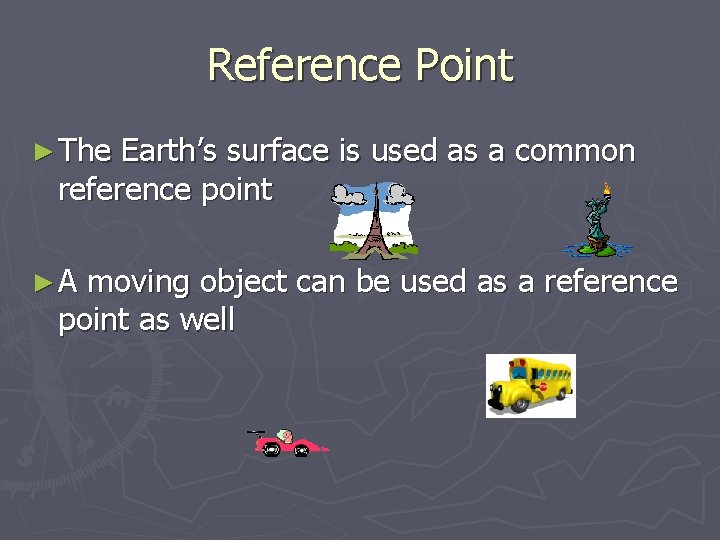Reference Point ► The Earth’s surface is used as a common reference point ►A moving object can be used as a reference point as well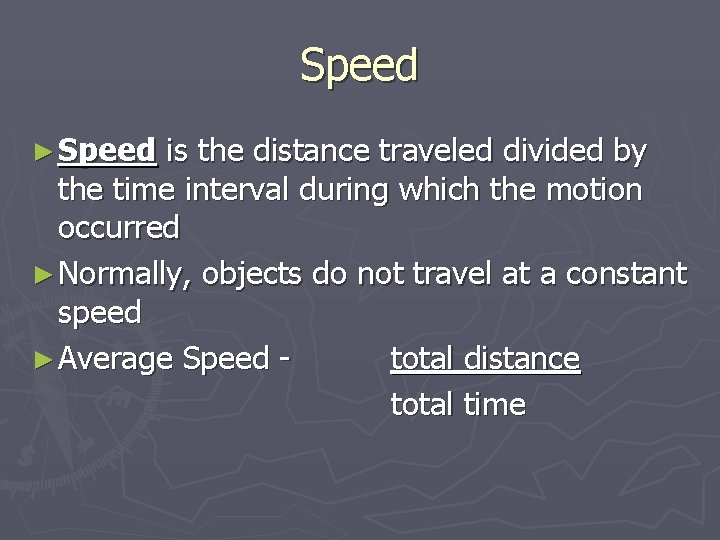Speed ► Speed is the distance traveled divided by the time interval during which the motion occurred ► Normally, objects do not travel at a constant speed ► Average Speed total distance total timeWhich Distance? ► Farmer Jones drives 6 miles down a straight road. She turns around and drives 4 miles back. What was her average speed for this trip if it took 1 hour?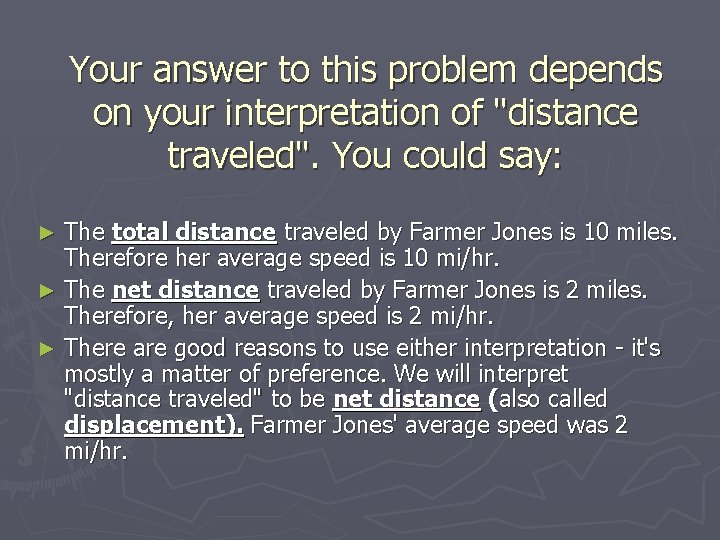Your answer to this problem depends on your interpretation of "distance traveled". You could say: The total distance traveled by Farmer Jones is 10 miles. Therefore her average speed is 10 mi/hr. ► The net distance traveled by Farmer Jones is 2 miles. Therefore, her average speed is 2 mi/hr. ► There are good reasons to use either interpretation - it's mostly a matter of preference. We will interpret "distance traveled" to be net distance (also called displacement). Farmer Jones' average speed was 2 mi/hr. ►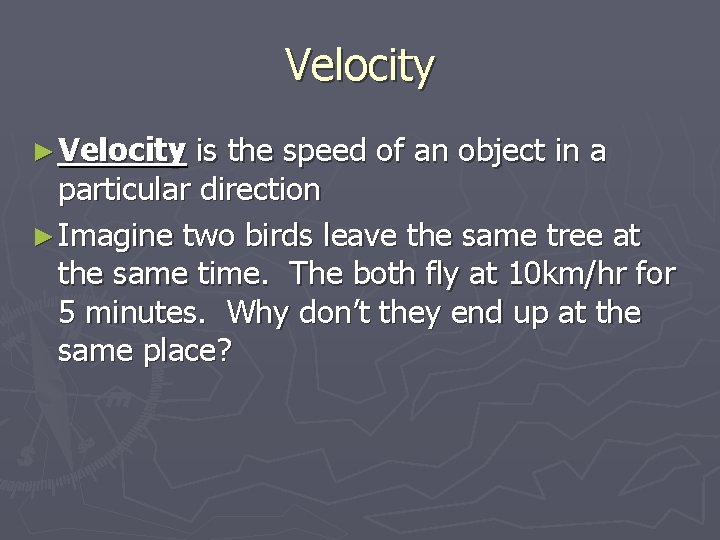Velocity ► Velocity is the speed of an object in a particular direction ► Imagine two birds leave the same tree at the same time. The both fly at 10 km/hr for 5 minutes. Why don’t they end up at the same place?Velocity ► Velocity appears to be very similar to speed, however, when describing the velocity of an object you need to provide a magnitude and a direction ► Magnitude – the speed of the object ► Direction – the direction the object is movingVelocity ► Ex) A bus has a velocity of 35 miles/hr heading west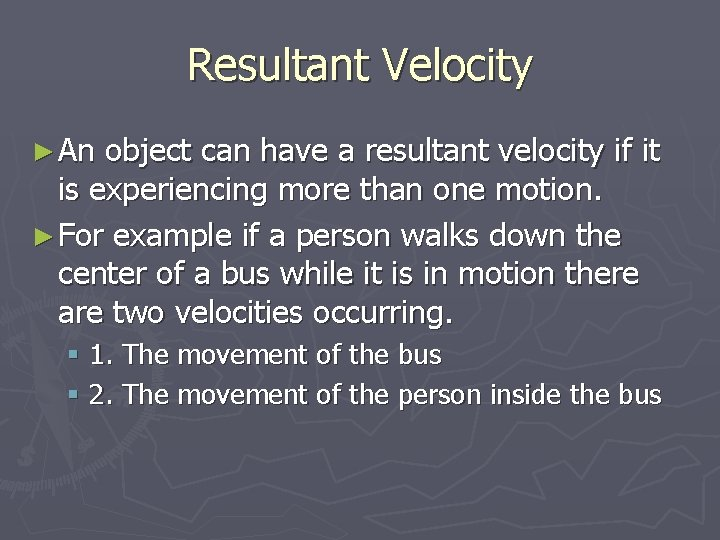Resultant Velocity ► An object can have a resultant velocity if it is experiencing more than one motion. ► For example if a person walks down the center of a bus while it is in motion there are two velocities occurring. § 1. The movement of the bus § 2. The movement of the person inside the bus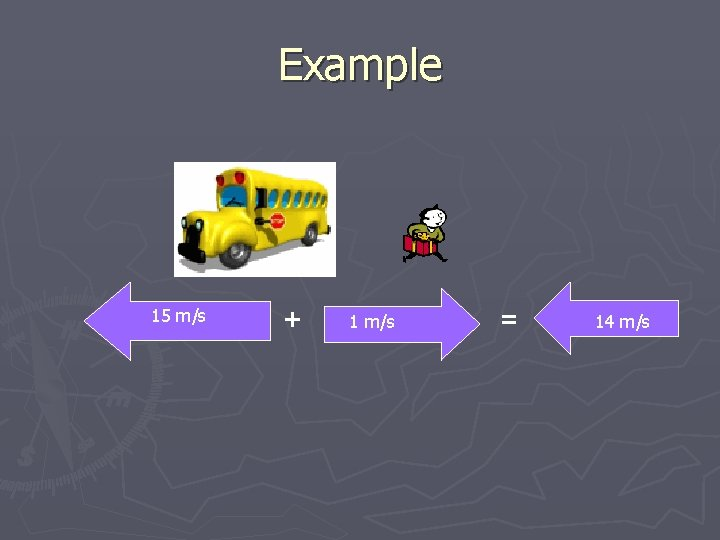Example 15 m/s + 1 m/s = 14 m/s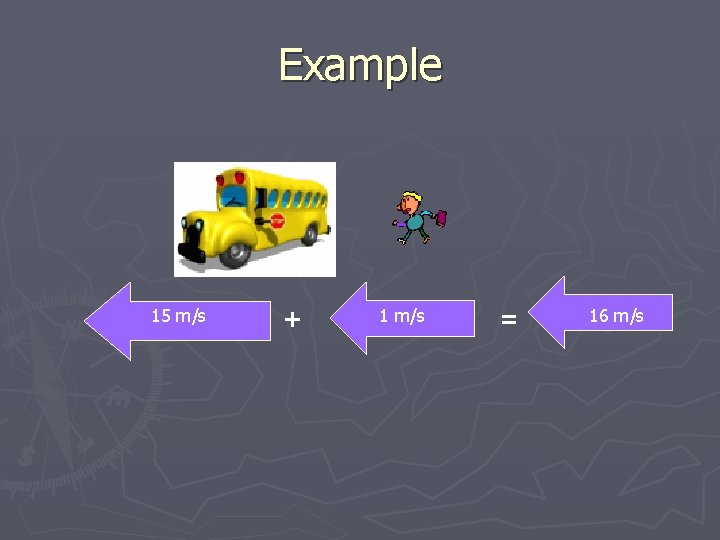Example 15 m/s + 1 m/s = 16 m/sAcceleration ► Acceleration is the rate at which velocity changes over time § An object accelerates if its speed, direction, or both change ► Average acceleration = final velocity – starting velocity time it takes to change velocity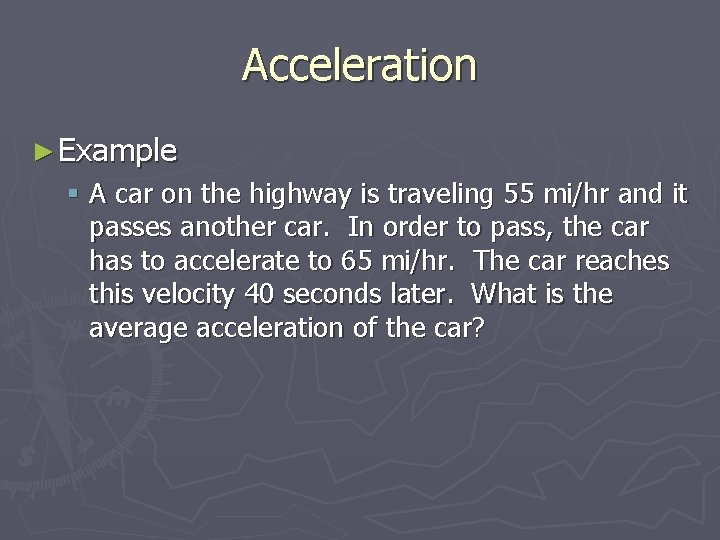Acceleration ► Example § A car on the highway is traveling 55 mi/hr and it passes another car. In order to pass, the car has to accelerate to 65 mi/hr. The car reaches this velocity 40 seconds later. What is the average acceleration of the car?Acceleration and Velocity ► http: //www. glenbrook. k 12. il. us/GBSSCI/PH YS/mmedia/kinema/avd. html ► As velocity increases, so does acceleration ► As velocity decreases, so does acceleration ► When direction changes, so does acceleration ► When there is a constant velocity, there is no acceleration